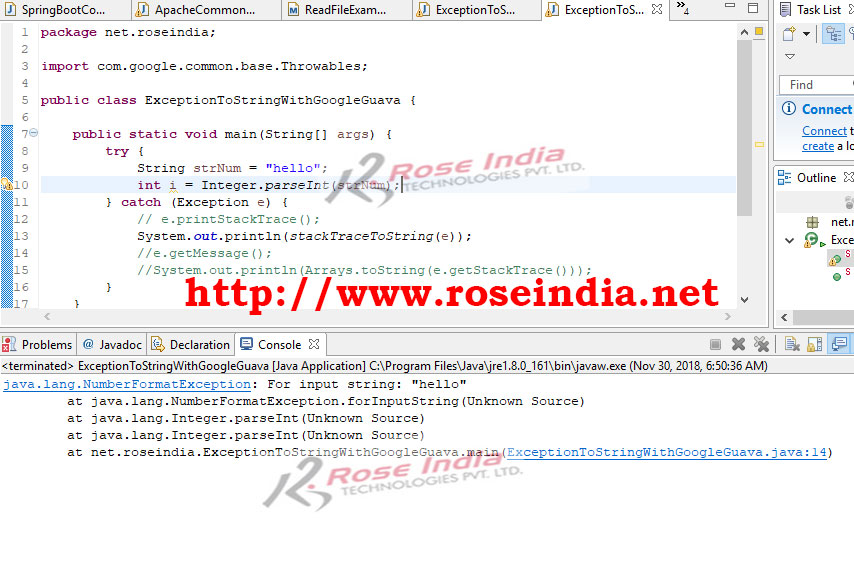Tutorials

# How to convert a stack trace to a string?

Learn how to convert a stack trace to a String which includes all the Stack Trace message?

Learn how to convert a stack trace to a String which includes all the Stack Trace message?

### Example of converting StackTrace to String in Java Program

In this tutorial we are going to learn different ways to convert StackTrace to String in Java program. This conversion is required if you want to save the message in custom log file for further analysis of log message. The e.getMessage() in Java program only returns small portion of the error, but if you want to see the detailed error then StackTrace will give you complete detail about the error in the program.

Here we are going to discuss about few methods which can be used for the conversion of StackTrack error message into String. You can use any of the following methods:

• Core Java Libraries
• Arrays class
• Apache commons stack trace to String

### Conversion of StackTrace with Java APIHere one example of converting StackTrace to String in Java:

```package net.roseindia;

import java.io.PrintWriter;
import java.io.StringWriter;

public class ExceptionToString {

public static void main(String[] args) {
try {
String strNum = "hello";
int i = Integer.parseInt(strNum);
} catch (Exception e) {
// e.printStackTrace();
System.out.println(stackTraceToString(e));
}
}

public static String stackTraceToString(Exception e) {
StringWriter sw = new StringWriter();
PrintWriter pw = new PrintWriter(sw);
e.printStackTrace(pw);
String sStackTrace = sw.toString();
return sStackTrace;
}
}
```

If you run the above program it will convert StackTrace to String and print following converted error message:

```java.lang.NumberFormatException: For input string: "hello"
at java.lang.NumberFormatException.forInputString(Unknown Source)
at java.lang.Integer.parseInt(Unknown Source)
at java.lang.Integer.parseInt(Unknown Source)
at net.roseindia.ExceptionToString.main(ExceptionToString.java:11)
```

In the above example code we have used StringWriter and PrintWriter classes to make a function which converts StrackTrace to String.

We have created following function for the conversation of StackTrace into String:

```public static String stackTraceToString(Exception e) {
StringWriter sw = new StringWriter();
PrintWriter pw = new PrintWriter(sw);
e.printStackTrace(pw);
String sStackTrace = sw.toString();
return sStackTrace;
}
```

This function can be used from another class for the conversion of error message into String.

### Google Guava Library for conversion of StackTrace into String

The Google Guava is utility library in Java which provides many classes and methods which can be used in general programming. In this tutorial we are going to use the Throwables.getStackTraceAsString(e) method for converting StackTrace into String in our Java program.

Method signature: static String getStackTraceAsString(Throwable throwable)

This method takes the Throwable object as parameter and then returns a string which includes result of toString() and full, recursive stack trace of throwable object. So, it returns complete error details in String format.

Here is complete example:

```package net.roseindia;

import java.io.PrintWriter;
import java.io.StringWriter;

public static void main(String[] args) {
try {
String strNum = "hello";
int i = Integer.parseInt(strNum);
} catch (Exception e) {
// e.printStackTrace();
System.out.println(stackTraceToString(e));
//e.getMessage();
}
}

public static String stackTraceToString(Exception e) {
String strStackTrace = Throwables.getStackTraceAsString(e);
return strStackTrace;
}
}
```

```<dependency>
<artifactId>guava</artifactId>
<version>21.0</version>
</dependency>
```

Apache maven is the easily method of managing dependency in Java projects. You can check more tutorials at Maven 3 Tutorials.

### With Arrays Class

Following code can also be used:

`import java.util.Arrays;`

After import Arrays class you can use following code for conversion:

`Arrays.toString(e.getStackTrace());`

But this code is returning all the error message in one line. For example if you can this code it will give you following output:

As you can see all the output is in one line.

### Apache commons stack trace to String

The Apache commons library can also be used to convert stack trace to String in Java. Here is an example of converting Stack Trace to String using Apache commons library:

```package net.roseindia;

import org.apache.commons.lang3.exception.ExceptionUtils;

public static void main(String[] args) {
try {
String strNum = "hello";
int i = Integer.parseInt(strNum);
} catch (Exception e) {
// e.printStackTrace();
System.out.println(stackTraceToString(e));
}
}

public static String stackTraceToString(Exception e) {
String strStacktrace = ExceptionUtils.getStackTrace(e);
return strStacktrace;
}
}
```

In this section we have explored various ways to convert Exception Stack Trace into String.

Here are more tutorials related to Exception handling in Java:

We have 1000's of Java programming tutorial on our website and all you can browser from the Java Tutorials index page. Our tutorials are organized into sections for easy navigation.